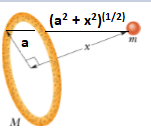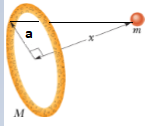# Problem: An object in the shape of a thin ring has radius a and mass M. A uniform sphere with mass m and radius R is placed with its center at a distance x to the right of the center of the ring, along a line through the center of the ring, and perpendicular to its plane (Figure 1).Part AWhat is the gravitational force that the sphere exerts on the ring-shaped object?Express your answer in terms of the variables a, M, m, R, x, and appropriate constants.

###### FREE Expert Solution

Newtons law of universal gravitation:

$\overline{){\mathbf{F}}{\mathbf{=}}\frac{{\mathbf{GM}}_{\mathbf{1}}{\mathbf{M}}_{\mathbf{2}}}{{\mathbf{R}}^{\mathbf{2}}}}$R = (a2 + x2)(1/2)

$\mathbit{F}\mathbf{=}\frac{\mathbf{G}\mathbf{M}\mathbf{m}}{\mathbf{\left(}{\mathbf{a}}^{\mathbf{2}}\mathbf{+}{\mathbf{x}}^{\mathbf{2}}\mathbf{\right)}}$

96% (190 ratings)###### Problem Details

An object in the shape of a thin ring has radius a and mass M. A uniform sphere with mass m and radius R is placed with its center at a distance x to the right of the center of the ring, along a line through the center of the ring, and perpendicular to its plane (Figure 1).Part A

What is the gravitational force that the sphere exerts on the ring-shaped object?

Express your answer in terms of the variables a, M, m, R, x, and appropriate constants.